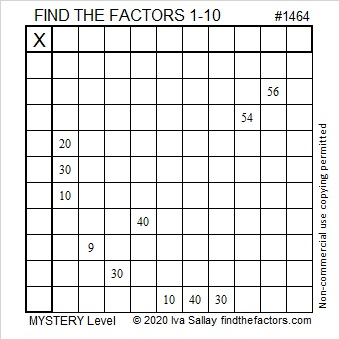# 1464 Alibis and a Mystery

Contents

### Today’s Puzzle:

You find clues 30, 30, 30, 54. Two of those 30’s claim to be 3 × 10.
When you find clues 40, 40, 56, you realize that at least one of the 40’s must be 4 x 10.

Can you believe the alibis the 30’s have just given you?
Can you put your detective skills together to figure out this mystery?### Factors of 1464:

• 1464 is a composite number.
• Prime factorization: 1464 = 2 × 2 × 2 × 3 × 61, which can be written 1464 = 2³ × 3 × 61
• 1464 has at least one exponent greater than 1 in its prime factorization so √1464 can be simplified. Taking the factor pair from the factor pair table below with the largest square number factor, we get √1464 = (√4)(√366) = 2√366
• The exponents in the prime factorization are 3,1 and 1. Adding one to each exponent and multiplying we get (3 + 1)(1 + 1) (1 + 1) = 4 × 2 × 2 = 16. Therefore 1464 has exactly 16 factors.
• The factors of 1464 are outlined with their factor pair partners in the graphic below.### More Facts about the Number 1464:

1464 is the hypotenuse of a Pythagorean triple:
264-1440-1464 which is 24 times (11-60-61).

OEIS.org alerts us that 1464 is a repdigit in two different bases:
It’s 1111 in BASE 11 because 11³ + 11² + 11¹ + 11º = 1464, and
it’s 888 in BASE 13 because 8(13² + 13¹ + 13º) = 8(183) =1464.

This site uses Akismet to reduce spam. Learn how your comment data is processed.